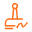SaaS公有云PC端APPSaaS免费版
SaaS VIP

1 电子签章

× 需自建平台

CA数字证书

× × × ×

× × × ×

× × × ×

× × × ×

× × × ×
2 审批流程

×

×

×

× ×

× ×

×

×

× ×

×

×
3 文件归档

× ×

× ×

× ×

× ×

× × × ×

× ×

× ×

× ×

× ×

× ×

× ×
4 文件模板

× ×

× ×

× ×

× ×

× ×
5 合同管理（选配）

× ×

× ×

× ×

× ×
6 客户管理（选配）

× ×

× ×

× ×

× ×AndroidIOS